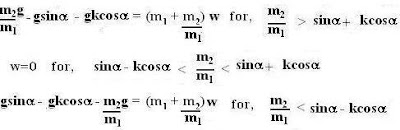## Monday, August 6, 2007

### Irodov Problem 1.64

This problem is actually an extension of problem 1.63 that has already been solved in the previous post. Hence, throughout this problem I shall refer to the equations and figures of problem 1.63.
Equations (5) and (7) can be used to determine the acceleration of the masses depending on whether the body is acceleratingdown or up as determined from conditions given by inequalities (6) and (8). Thus, we can write,and thus we can re-write them as,#### 1 comment: